Next: Phase relationships between channels Up: Fourier analysis and resynthesis Previous: Timbre stamping (classical vocoder)   Contents   Index

Phase

So far we have operated on signals by altering the magnitudes of their windowed Fourier transforms, but leaving phases intact. The magnitudes encode the spectral envelope of the sound. The phases, on the other hand, encode frequency and time, in the sense that phase change from one window to a different one accumulates, over time, according to frequency. To make a transformation that allows independent control over frequency and time requires analyzing and reconstructing the phase.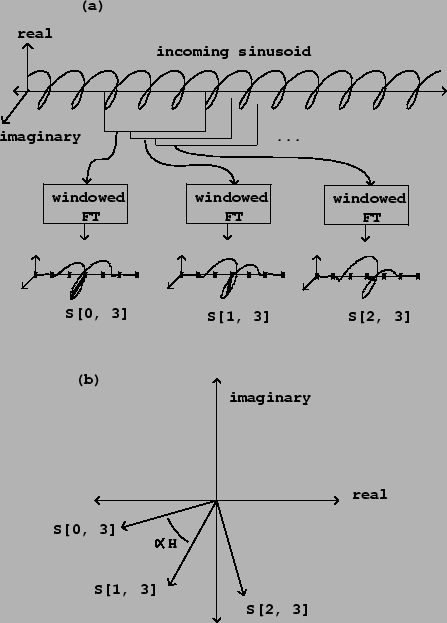In the analysis/synthesis examples of the previous section, the phase of the output is copied directly from the phase of the input. This is appropriate when the output signal corresponds in time with the input signal. Sometimes time modifications are desired, for instance to do time stretching or contraction. Alternatively the output phase might depend on more than one input, for instance to morph between one sound and another.

Figure 9.10 shows how the phase of the Fourier transform changes from window to window, given a complex sinusoid as input. The sinusoid's frequency is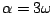, so that the peak in the Fourier transform is centered at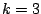. If the initial phase is, then the neighboring phases can be filled in as: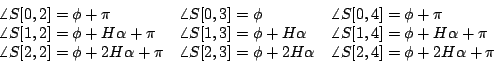This gives an excellent way of estimating the frequency: pick any channel whose amplitude is dominated by the sinusoid and subtract two successive phase to get: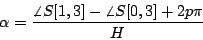where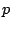is an integer. There arepossible frequencies, spaced by. If we are using an overlap of 4, that is,, the frequencies are spaced by. Happily, this is the width of the main lobe for the Hann window, so no more than one possible value ofcan explain any measured phase difference within the main lobe of a peak. The correct value ofto choose is that which gives a frequency closest to the nominal frequency of the channel,.

When computing phases for synthesizing a new or modified signal, we want to maintain the appropriate phase relationships between successive resynthesis windows, and also, simultaneously, between adjacent channels. These two sets of relationships are not always compatible, however. We will make it our first obligation to honor the relations between successive resynthesis windows, and worry about phase relationships between channels afterward.

Suppose we want to construct theth spectrum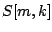for resynthesis (having already constructed the previous one, number). Suppose we wish the phase relationships between windowsandto be those of a signal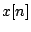, but that the phases of window numbermight have come from somewhere else and can't be assumed to be in line with our wishes.To find out how much the phase of each channel should differ from the previous one, we do two analyses of the signal, separated by the same hop sizethat we're using for resynthesis: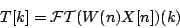Figure 9.11 shows the process of phase accumulation, in which the output phases each depend on the previous output phase and the phase difference for two windowed analyses of the input. Figure 9.12 illustrates the phase relationship in the complex plane. The phase of the new outputshould be that of the previous one plus the difference between the phases of the two analyses:Here we used the fact that multiplying or dividing two complex numbers gives the sum or difference of their arguments.

If the desired magnitude is a real number, then we should setto:The magnitudes of the second and third terms cancel out, so that the magnitude ofreduces to; the first two terms are real numbers so the argument is controlled by the last term.

If we want to end up with the magnitude from the spectrum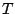as well, we can setand simplify:SubsectionsNext: Phase relationships between channels Up: Fourier analysis and resynthesis Previous: Timbre stamping (classical vocoder)   Contents   Index
Miller Puckette 2006-12-30